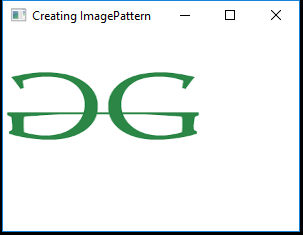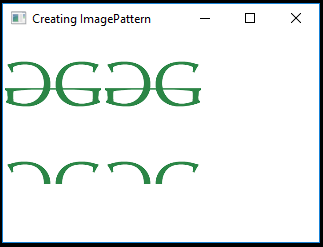# JavaFX | ImagePattern Class

ImagePattern is a part of JavaFX. This class is used to fills a shape with an image pattern. A user may specify the anchor rectangle, which defines the position, width, and height of the image relative to the upper left corner of the shape. If the shape extends out of the anchor rectangle, the image is tiled.

Constructors of the class:

1. ImagePattern(Image i): Creates a new instance of ImagePattern with the specified image.
2. ImagePattern(Image i, double x, double y, double width, double height, boolean prop): Creates an image with specified x, y coordinate, a defined width and height and whether it is proportional or not.

Commonly Used Methods:

Method Explanation
getHeight() Returns the height of the image pattern.
getWidth() Returns the width of image pattern.
getImage() Returns the image of the image pattern.
getX() Returns the X origin of the anchor rectangle.
getY() Returns the Y origin of the anchor rectangle.
isOpaque() Returns whether the paint is completely opaque or not.
isProportional() Returns whether the start and end locations is completely proportional or not.

Below programs illustrate the use of ImagePattern Class:

1. Java Program to create a ImagePattern from a image and apply it to the rectangle: In this program we will create a ImagePattern named image_pattern from a image. Import the image using a FileInputStream. Add this image_pattern to the rectangle using the setFill() function. Create a VBox and add the rectangle to the vbox. Add the vbox to the scene and add the scene to the stage. Call the show() function to display the results.

 `// Java Program to create a ImagePattern from ` `// a image and apply it to the rectangle ` `import` `javafx.application.Application; ` `import` `javafx.scene.Scene; ` `import` `javafx.scene.control.*; ` `import` `javafx.scene.layout.*; ` `import` `javafx.event.ActionEvent; ` `import` `javafx.event.EventHandler; ` `import` `javafx.scene.control.Label; ` `import` `javafx.stage.Stage; ` `import` `javafx.scene.image.*; ` `import` `java.io.*; ` `import` `javafx.scene.paint.*; ` `import` `javafx.scene.shape.*; ` ` `  `public` `class` `ImagePattern_1 ``extends` `Application { ` ` `  `    ``// launch the application ` `    ``public` `void` `start(Stage s) ``throws` `Exception ` `    ``{ ` ` `  `        ``// set title for the stage ` `        ``s.setTitle(``"Creating ImagePattern"``); ` ` `  `        ``// create a input stream ` `        ``FileInputStream input = ``new` `FileInputStream(``"D:\\GFG.png"``); ` ` `  `        ``// create a image ` `        ``Image image = ``new` `Image(input); ` ` `  `        ``// create ImagePattern ` `        ``ImagePattern image_pattern = ``new` `ImagePattern(image); ` ` `  `        ``// create a Rectangle ` `        ``Rectangle rect = ``new` `Rectangle(``100``, ``100``, ``200``, ``150``); ` ` `  `        ``// set fill for rectangle ` `        ``rect.setFill(image_pattern); ` ` `  `        ``// create a VBox ` `        ``VBox vbox = ``new` `VBox(rect); ` ` `  `        ``// create a scene ` `        ``Scene sc = ``new` `Scene(vbox, ``200``, ``200``); ` ` `  `        ``// set the scene ` `        ``s.setScene(sc); ` ` `  `        ``s.show(); ` `    ``} ` ` `  `    ``public` `static` `void` `main(String args[]) ` `    ``{ ` ` `  `        ``// launch the application ` `        ``launch(args); ` `    ``} ` `} `

Input Image:Output:2. Java Program to create an ImagePattern form an image, set the x, y coordinate, its height and width, and whether it is proportional or not and apply it to the rectangle: In this program we will create an ImagePattern named image_pattern from an image. Import the image using a FileInputStream. Specify the x, y coordinates of the anchor rectangle, its height, width and whether it is proportional or not bypassing the values as arguments of the constructor of ImagePattern. Add this image_pattern to the rectangle using the setFill() function. Create a VBox and add the rectangle to vbox. Add the vbox to the scene and add the scene to the stage. Call the show() function to display the results.

 `// Java Program to create an ImagePattern form an image,  ` `// set the x, y coordinate, its height and width, and  ` `// whether it is proportional or not and apply it to ` `// the rectangle ` `import` `javafx.application.Application; ` `import` `javafx.scene.Scene; ` `import` `javafx.scene.control.*; ` `import` `javafx.scene.layout.*; ` `import` `javafx.event.ActionEvent; ` `import` `javafx.event.EventHandler; ` `import` `javafx.scene.control.Label; ` `import` `javafx.stage.Stage; ` `import` `javafx.scene.image.*; ` `import` `java.io.*; ` `import` `javafx.scene.paint.*; ` `import` `javafx.scene.shape.*; ` ` `  `public` `class` `ImagePattern_2 ``extends` `Application { ` ` `  `    ``// launch the application ` `    ``public` `void` `start(Stage s) ``throws` `Exception ` `    ``{ ` ` `  `        ``// set title for the stage ` `        ``s.setTitle(``"Creating ImagePattern"``); ` ` `  `        ``// create a input stream ` `        ``FileInputStream input = ``new` `FileInputStream(``"D:\\GFG.png"``); ` ` `  `        ``// create a image ` `        ``Image image = ``new` `Image(input); ` ` `  `        ``// create ImagePattern ` `        ``ImagePattern image_pattern = ``new` `ImagePattern(image, ``100``, ``100``, ` `                                                     ``100``, ``100``, ``false``); ` ` `  `        ``// create a Rectangle ` `        ``Rectangle rect = ``new` `Rectangle(``100``, ``100``, ``200``, ``150``); ` ` `  `        ``// set fill for rectangle ` `        ``rect.setFill(image_pattern); ` ` `  `        ``// create a VBox ` `        ``VBox vbox = ``new` `VBox(rect); ` ` `  `        ``// create a scene ` `        ``Scene sc = ``new` `Scene(vbox, ``200``, ``200``); ` ` `  `        ``// set the scene ` `        ``s.setScene(sc); ` ` `  `        ``s.show(); ` `    ``} ` ` `  `    ``// Main Method ` `    ``public` `static` `void` `main(String args[]) ` `    ``{ ` ` `  `        ``// launch the application ` `        ``launch(args); ` `    ``} ` `} `

Input Image:Output:Note: The above programs might not run in an online IDE please use an offline compiler.

Attention reader! Don’t stop learning now. Get hold of all the important DSA concepts with the DSA Self Paced Course at a student-friendly price and become industry ready.

My Personal Notes arrow_drop_upCheck out this Author's contributed articles.

If you like GeeksforGeeks and would like to contribute, you can also write an article using contribute.geeksforgeeks.org or mail your article to contribute@geeksforgeeks.org. See your article appearing on the GeeksforGeeks main page and help other Geeks.

Please Improve this article if you find anything incorrect by clicking on the "Improve Article" button below.

Article Tags :
Practice Tags :

Be the First to upvote.

Please write to us at contribute@geeksforgeeks.org to report any issue with the above content.